×

Search anything:

# Purpose of different layers in a Deep Learning Model

#### Machine Learning (ML)Get this book -> Problems on Array: For Interviews and Competitive Programming

In this article, we have explored the significance or purpose or importance of each layer in a Machine Learning model. Different layers include convolution, pooling, normalization and much more. For example: the significance of MaxPool is that it decreases sensitivity to the location of features.

We will go through each layer and explore its significance accordingly.

Layers are the deep of deep learning!

# Layers

This is the highest level building block in deep learning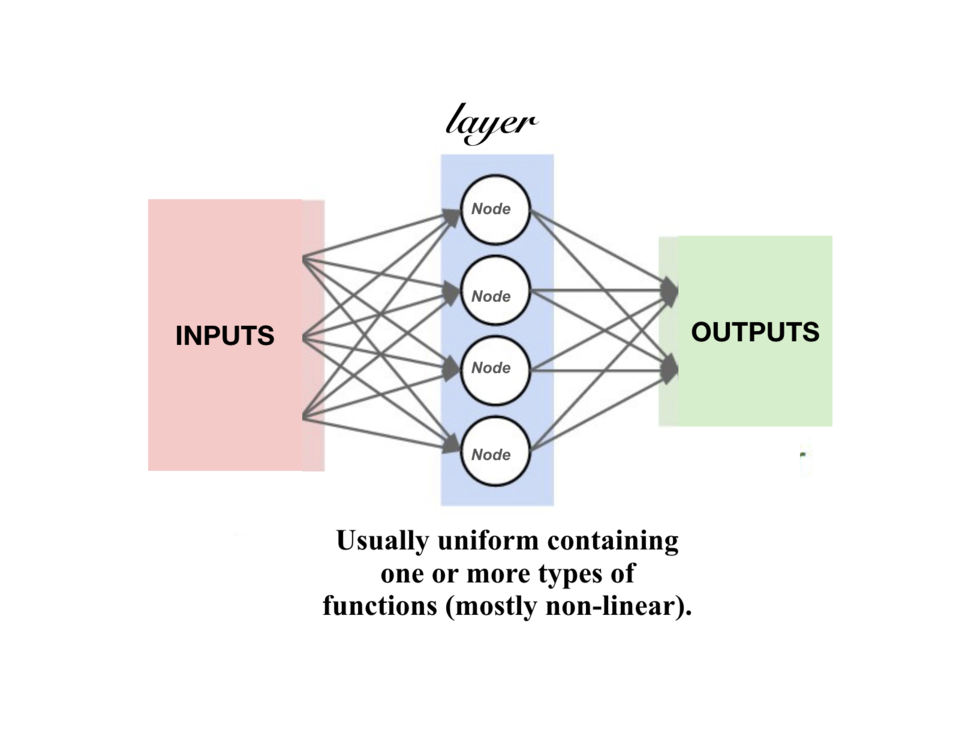Layers are made up of NODES, which take one of more weighted input connections and produce an output connection.
They're organised into layers to comprise a network.

Many such layers, together form a Neural Network, i.e. the foundation of Deep Learning.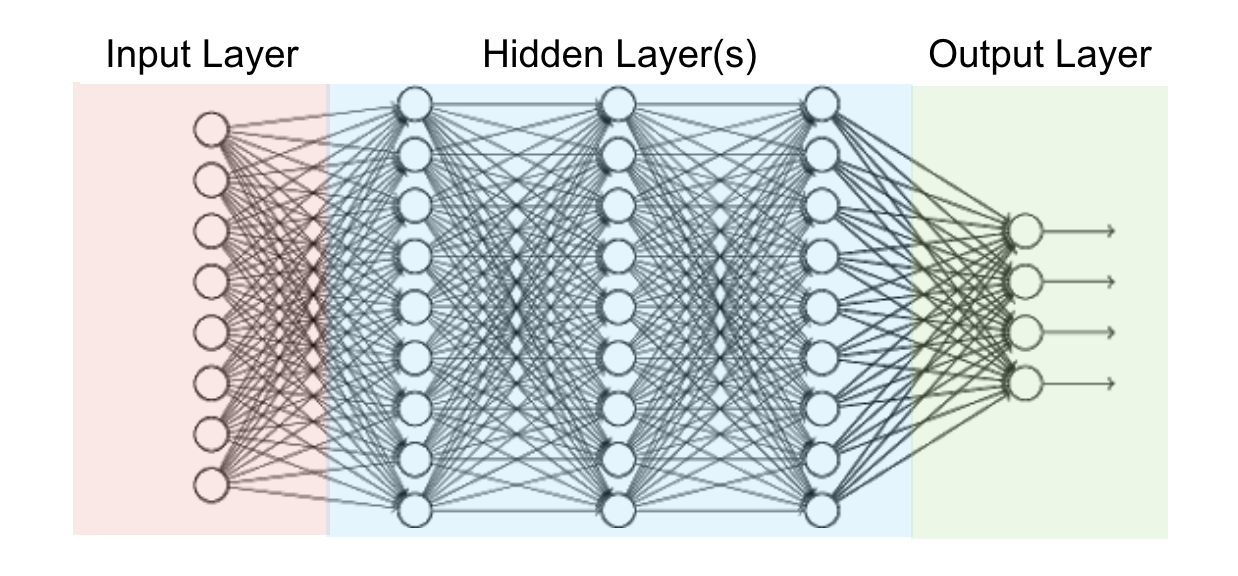By depth, we refer to the number of layers.

A variety of functions are performed by a variety of layers, each layer possesses its own utility and significance.

• Input layer - This is the layer that receives data.
• Normalisation layer - It scales input data to suitable intervals such that bias shall be removed.
• Convolutional layer - This applies filters to the input such that the features maybe detected along with their locations.
• Pooling layer - It decreases sensitivity to features, thereby creating more generalised data for better test results.
• Activation layer - This applies mathematical functions f(x) to input layer, such that the ability of learning something complex and interesting is developed.
• Dropout layer - This layer nullifies certain random input values to generate a more general dataset and prevent the problem of overfitting.
• Output layer - This is the layer that produces results, devised by our neural network.

It has been mentioned above in a particular flow, not particular to any network though, but for understanding point of view.

1. Read input through the input layer.
2. Normalise the input for efficient working (this maybe done within the input layer as well)
3. Convolute the data to identify features. Some datasets may have predefined features. Different datasets maybe convoluted to different degrees.
4. Pooling layer maybe introduced to decrease sensitivity to features found, making it more generalized.
5. Activation layer(s) maybe introduced to bring out results of significance.
6. In case of overfitting, dropout layers maybe introduces in between the code, so as to make the dataset more generalized
7. Finally, output layer shall provide necessary results.

You see, every layer plays a pivotal role! Below, let's cover them in detail!

## Input Layer

This is the most fundamental of all layers, as without an input layer a neural network cannot produce results. There is no point of any algorithm where no input may be fed.
They are of various kinds depending on the type of input, as follows

• 2-D image
• 3-D image
• Sequenced data input
• et cetera

Input layers might as well perform certain functions which enable smoother functioning of the neural network such as:

Normalisation is an approach applied during data preparation to change values of numeric columns in a data set to use a common scale when features in the data have different ranges.

• It maintains the contribution of each feature, and omits bias due to numeric value.
• It makes optimisation faster.

Layer normalisation normalises input across the features.

## Convolutional Layers

These are the building blocks of Convolutional Neural Networks.

In simplest terms,
It is the application of a filter to an input.

The same filter after being reapeatedly applied to the input may result in a map of activations which is known as feature map, it indicates the locations and strength of detected features in the input, such as an image.

Example :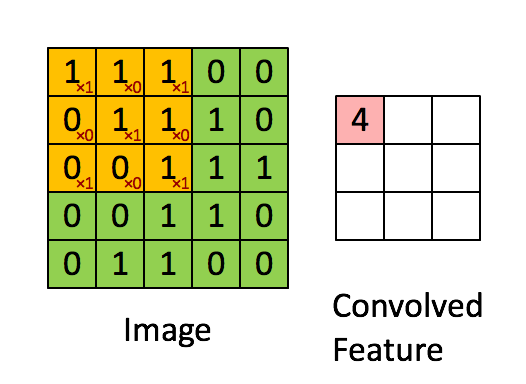These filters may be handcrafted for various feature detection such as

• Edge detection
• Object detection
• et cetera

These are detected by means of a feature map formed.

Subsequent connected layers together form a functional unit, the basis of computer vision!

Example: Below is number detection, on the left shows how subsequent layers are developing different feature maps, and on the bottom right we have the input and on the top right the Output.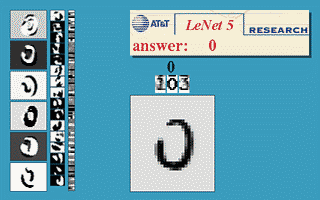The learned features during training of Convolutional Neural Networks also hold enormous power when it comes to pertaining to a specific problem.

These layers may fundamentally too, be of various kinds

• Convolution 2D/3D layer - It applies sliding convolutional filters to the input.
• Grouped Convolution 2D layer - It applies sliding cuboidal convolution filters to three-dimensional input.
• Transposed Convolution 2D/3D layer - It separates the input channels into groups and applies sliding convolutional filters on them.

## Activation Layer

We apply activation function f(x) so as to make the network powerful and add ability to it to learn something complex and complicated and represent non-linear complex arbitrary function mappings between input and output.

We can uniquely identify the layer by corresponding activation functions.
Few of them are described below.

#### Sigmoid

f(x) = 1 / 1 + exp(-x)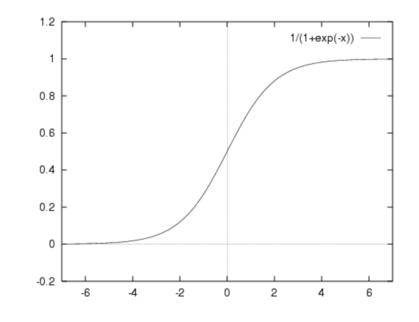• It has smooth gradient, preventing sudden jump in output values

• Gives output values between 0 and 1. It enables clear predictions

• Vanishing Gradient Problem - For very high and very low values no changes in prediction shall be observed. Hence, the network refuses to learn further, or becomes too slow to reach an accurate prediction.

#### TanH / Hyperbolic Tangent

f(x) = tanh(x) = ( (2 / 1 + exp(-2x)) - 1 )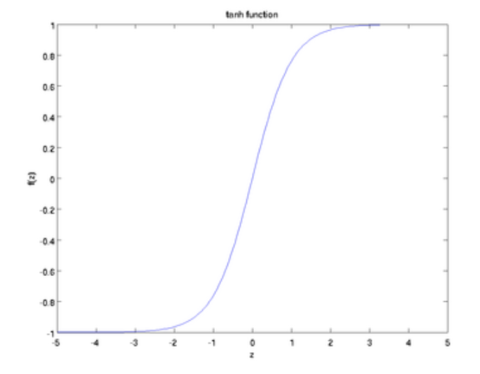• It is zero centered, and makes it easier to model strongly negative, strongly positive and neutral.

It is similar to the sigmoid function. It is a scaled sigmoid function.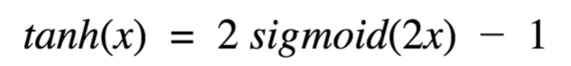#### ReLu Layer

f(x) = max(0,x)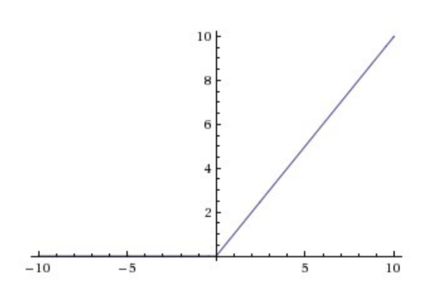• It is computationally efficient and allows the network to converge very quickly

• It is non-linear although it looks like a linear function, ReLU has a derivative function and allows for backpropagation

• The Dying ReLU problem - When inputs approach zero, or are negative, the gradient of the function becomes zero, the network cannot perform backpropagation and cannot learn.

Other variations exist to be of better use such as Parametric ReLU layer, Leaky ReLU layer, Clipped ReLU layer, eLU layer, preLU layer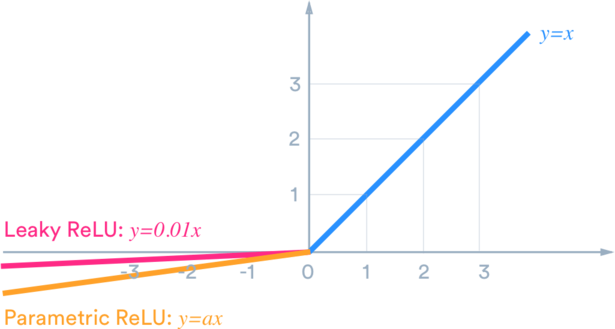When building a model and training a neural network, the selection of activation function is critical, and you may experiment with a lot of them!

## Normalisation and Dropout layer

These layers are usually included to deal with the problem of overfitting, i.e. when a neural network models the test set with great perfection, but only works well for the test set and not any general test case.

#### Crop layer

It crops the volume to the size of input feature map.
Eg. 2DCroplayer, 3DCroplayer

It is different from the resize layer which resizes the shape of the input according to the input feature map. It crops the image.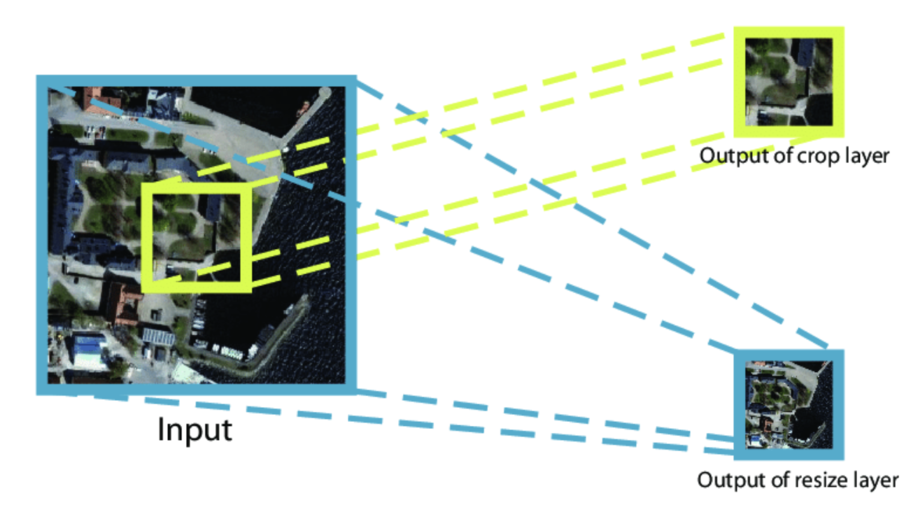#### Dropout layer

It randomly nullifies input elements. Eg. If elements represent probability, it must randomly sets input elements to zero.
Dropout prevents overfitting due to a layer's "over-reliance" on a few of its inputs and improves generalization.

#### Normalisation layer

As prescribed earlier, while discussing input layers, it is a method to scale data into suitable interval.

It generally speeds up learning and yields faster convergence, and thus are preferably used while taking input.
They can be introduced in between, as per programmer's approach too.

Examples include batch normalization, layer normalization, cross channel normalisation layer et cetera.

## Pooling layers

Convolutional layers, bring forth the "presence" of features and so they tend to be sensitive to location of features and such sensitivity gives rise to bias.
Pooling layers reduce the sensitivity to location of features.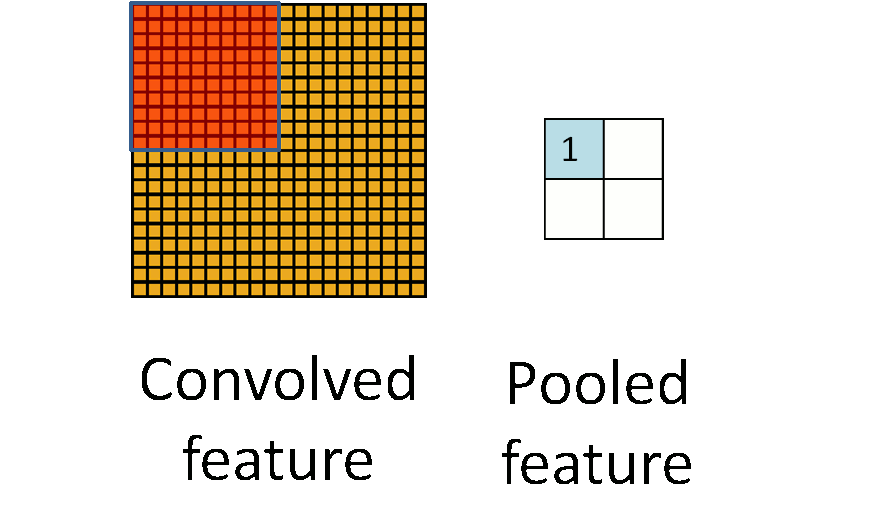Pooling maybe done by different kinds of layers, some of them are :

#### Average Pooling

An average pooling layer down-samples by dividing the input into sub-regions known as pooling regions and computing the average values for each region.

This layer could be made for 2D input, 3D input etc. taking average of region or by computing the mean of the height and width dimensions of the input.

#### Max Pooling

A max pooling layer down-samples by dividing the input into sub-regions known as pooling regions and computing the maximum for each region.

The sub-region maybe rectangular for a 2D input layer, cuboidal for a 3D input layer. It could also perform down-sampling by computing the maximum of the height and width dimensions of the input.

Note- As pooling layers exist, unpooling layers may also be devised, and used while working with some dataset.

## Customised Layer

The possibilities with layers are enormous. That is what brings glory to neural networks, and is the key to enhancement and enrichment.
Above are some of the standard layers, you could also

• Use a combination of them
• Build a new layer with new formulae and functions
• Use more purpose-specific layers from external libraries to get the job done!

## Question

#### Which of these layers you cannot find in a CNN ?

Convolutional Layer
Pooling layer
ReLu Layer
They all may exist
There are no particular rules to which layer goes where and images are ultimately a numeric matrix and all numeric calculations are possible on it. That is how we interact with them!

And there you have it, you've have been briefed through the significance of various famous layers and their impact on your neural networks!
Now you may explore more as you better understand your neural network and can enhance your efficiency!!#### Priyanshi Sharma

Priyanshi Sharma has been an Intern at Google in 2021 | Computer Science student at National Institute of Technology, Raipur | 2020 Intern at OpenGenus | Author of "Problems in AI" |

Purpose of different layers in a Deep Learning Model